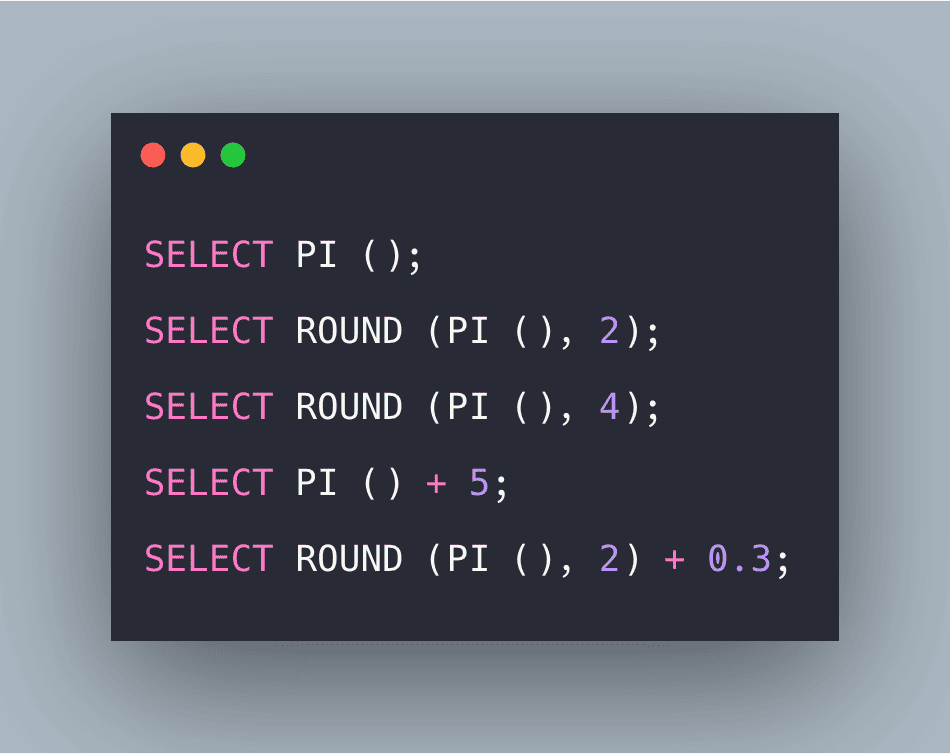# SQL PI Function: The Complete Guide

0
7SQL PI function is used for returning the mathematical value of the constant π (pie). The PI() function returns the value of PI.

## SQL PI

PI is a mathematical constant number to represent the ratio of a circle’s circumference to its diameter. The value of Pi is approximately equal to 3.14159. It is an irrational number. 22/7 is also another form of Pi value. In computer programming, we usually use this as a constant number as 3.1415 or with more decimal values.

### Syntax

```SELECT PI ();
```

Here, the PI function will return the mathematical value.

### Examples

#### Query 1

```SELECT PI ();
```

#### Output

`3.14159265358979`

#### Query 2

```SELECT ROUND (PI (), 2);
```

`3.14`

#### Explanation

Here, as the round function is used, which returned the value correct to 2 decimal places.

#### Query 3

```SELECT ROUND (PI (), 4);
```

#### Output

```3.1416
```

#### Explanation

Here, as the round function is used, which returned the value correct to 2 decimal places.

#### Query 4

`SELECT PI () + 5;`

#### Output

```8.14159265358979
```

#### Explanation

Here, arithmetic operations are performed between the value returned by PI() function and the value 5.

#### Query 5

```SELECT ROUND (PI (), 2) + 0.3;
```

```3.44
```

#### Explanation

Here, arithmetic operations are performed between the value returned by the PI () function, and the value 0.3 further the result is rounded to 2 decimal places.